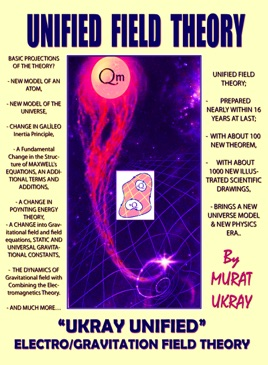## Publisher Description

"UKRAY" - UNIFIED FIELD THEORY -
- A New Unification Theory on Electromagnetic Gravitation-

PREFACE

“This study which aims to prove that all forces and laws of physics exist in a single unified structure at the Starting and Ending moment of the Universe analyzes all laws of physics within the framework of a unified structure from Newton Mechanics to Quantum Theory, Einstein Relativity to modern 11-dimensional Super string theory. The study may also be considered as a "MODERN ERA PRINCIPIA" since it was started to be written in about 300 years (early 2007) after the publication of the great study of Newton named "PRINCIPIA" (1703-1707) on the topic of gravity theories. The volume includes SEVEN CHAPTERS in the form of SEVEN different articles which follow each other and make clear the subject when they are read consecutively. In addition, FOUR additional chapters in the form of APPENDIXES in nature of FUNDAMENTALS OF MATHEMATICS were also included at the end of the volume for readers who have a less degree of technical knowledge about the topic…

THIS THEORY, GETS THESE QUESTIONS INTO;

- A CHANGE into Gravitational field and field equations, STATIC AND UNIVERSAL GRAVITATIONAL CONSTANTS,
- THE DYNAMICS OF Gravitational field with Combining the Electromagnetics Theory.
- THE VELOCITY OF LIGHT COULD BE EXCEEDED?
THIS THEORY WAS PREPARED AS A CONSEQUENCE OF APPROXIMATELY 16 YEARS STUDY,
- WHOLE "666" PAGE
- INCLUDES ABOUT 100 THEOREMS,
- AND 1000 ILLUSTRATED DRAWINGS,
- ASSERTS THE NEW PHYSICS OF THE UNIVERSE.
AND MUCH MORE…

"I imagined the situation of a mass falling towards the singularity point in a blackhole singularity in electrodynamic gravity conditions for some relative structures in the electromagnetic theory which is the most important and understandable theory in the classical physics I had comprehensive knowledge in my last years of my undergraduate term of the academic life (in about 2000) in an article of Faraday on the topic of the law of induction I had incidentally seen while I was examining the existing physics literature in the faculty's library. I wondered if the law of induction in a circular conducting wire differently perceived according to an observer in the train and the one on the land in the special relativity of Einstein may occur by the increase and decrease of mass during the course of falling to singularity in this blackhole and may create an electromagnetic gravity wave and a magnetic charge current which would decrease the impact of gravitation in parallel to this.

This oriented me to a series of researches to study and create this theory for years and then directed me to create a unified electromagnetic gravity theory composed of SEVEN ARTICLES in total I will submit here in order and step by step. Even though the theory includes a deductive mathematical approach, tensor calculation and geometric modellings, I will give solutions of Einstein-Maxwell Equations with a different mathematical 4x4 Pauli-Dirac Spinors and Tensor calculation construction in direction of closed extra dimension of the space (5 Dimension Effect)

What Does the Theory Tell?

{Short Abstract and Philosophy of the Theory}

The THEORY summarizes the general and simple mathematical description of the universe in the form of general conclusion items and forecasts the followings;

Basic Projections of the Theory?

- NEW MODEL OF AN ATOM,
- NEW MODEL OF THE UNIVERSE,
- CHANGE IN GALILEO Inertia Principle,
- A Fundamental Change in the Structure of MAXWELL's EQUATIONS, AN ADDITIONAL TERMS AND ADDITIONS,
- A CHANGE IN POYNTING ENERGY THEORY,
- A NEW ATOMIC MODEL,
- A NEW UNIVERSE MODEL,
- CHANGE IN GALILEO'S PRINCIPLE OF INERTIA,
- A FUNDEMENTAL CHANGE AND AN ADDITIONAL TERM IN THE STRUCTURE IF MAXWELL EQUATIONS,
- A CHANGE IN STATIC FIELD EQUATIONS OF THE GRAVITY FIELD AND IN THE UNIVERSAL GRAVITY CONSTANT.
- CHANGE IN POYNTING ENERGY THEOREM,
- HOW CAN THE VELOCITY OF LIGHT BE EXCEEDED?